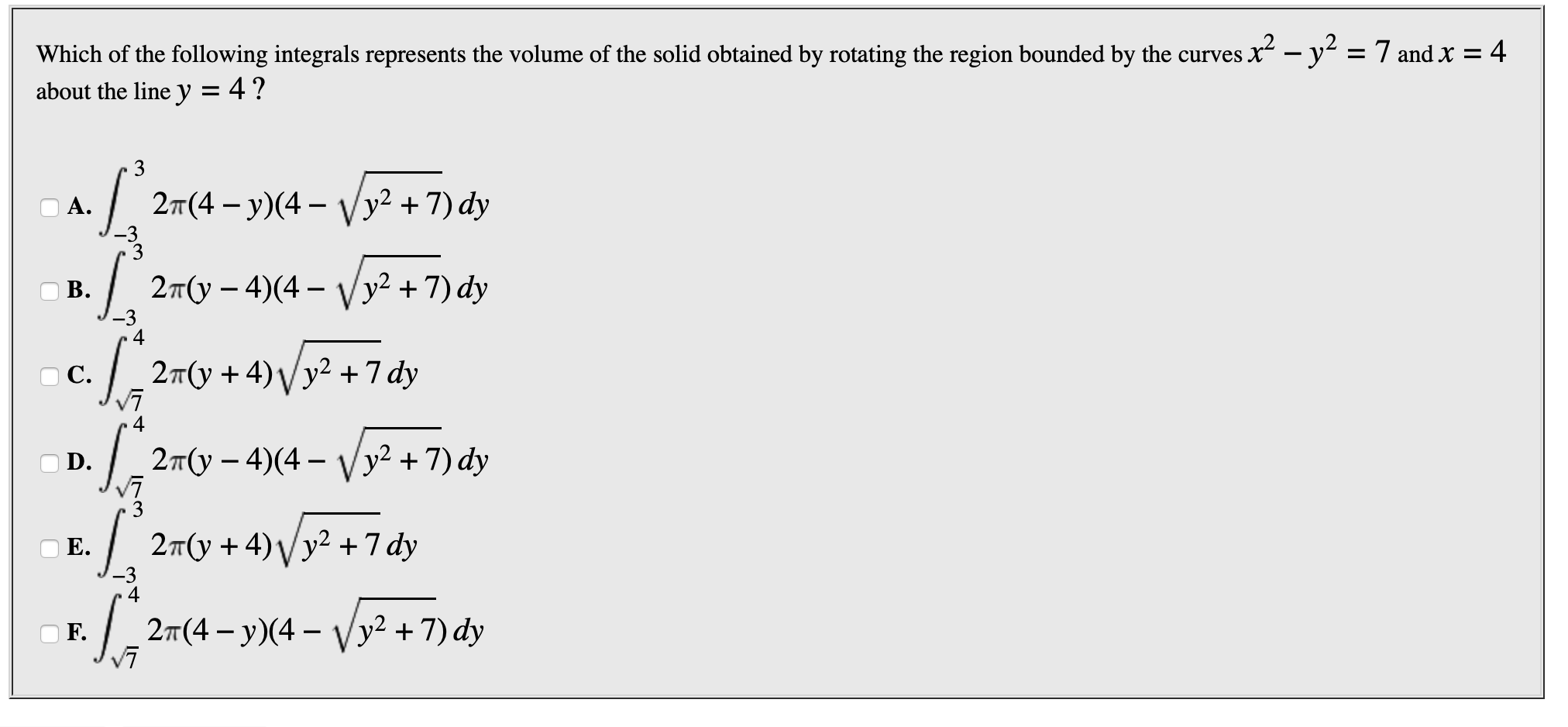# Which of the following integrals represents the volume of the solid obtained by rotating the region bounded by the curves xabout the line y = 4?y = 7 and X = 42T(4-y)(4Vy2 +7) dyА.-33.2r(y-4) ( 4- Vy2 +7) dy-32т(у + 4) у у2 +7 dyC4. 2T(y-4)(4- Vy2 +7) dy.| 2T(y + 4)y2 7 dy-342т (4 — у)(4 — уу2 + 7) dyVF.

Question
14 views

problem in photo attachedhelp_outlineImage TranscriptioncloseWhich of the following integrals represents the volume of the solid obtained by rotating the region bounded by the curves x about the line y = 4? y = 7 and X = 4 2T(4-y)(4 Vy2 +7) dy А. -3 3 .2r(y-4) ( 4- Vy2 +7) dy -3 2т(у + 4) у у2 +7 dy C 4 . 2T(y-4)(4- Vy2 +7) dy .| 2T(y + 4)y2 7 dy -3 4 2т (4 — у)(4 — уу2 + 7) dy V F. fullscreen
check_circle

Step 1

Refer to the question we need to find the volume of the solid obtained by rotating the region bounded by the provided curves about the line y=4.

Step 2

Now sketch the graph of the provided data as,

Step 3

Use the Shell Method to find the volume w...

### Want to see the full answer?

See Solution

#### Want to see this answer and more?

Solutions are written by subject experts who are available 24/7. Questions are typically answered within 1 hour.*

See Solution
*Response times may vary by subject and question.
Tagged in

### Calculus